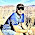## How to

When solving these problems, learning by doing is much better than learning by reading. I encourage to you read only as far in the solution as you need, then trying to solve the problem. If you get stuck, try reading a little further. And of course, let me know if you find a better solution!

## Sunday, February 12, 2012

### Print duplicated values in an array

Solution:  Given an array a which contains numbers only numbers between 1-99, print the duplicated values.  Discuss time/space tradeoffs.

void printDuplicates(int a[]){
...
}

Well, this problem seems pretty straight forward, but like most interview questions, it's designed to show that: 1) you can do the straight forward, and 2) you can understand trade-offs and how to do more complicated programming.

We can go through the array and make a note each time that we encounter a number.  If we encounter it again, we can then print it out as it's a duplicate.

Let's code it up and use a simple array, where the number we're tracking is the same as the array index to keep track of whether we have seen a number before.

void printDuplicates(int a[]){

int i;

//zero out the array
for (i=0; i < 100; i++)

//now, iterate through a and mark that we have seen a
//number, or print it if we have already.
//Watch out for off by one errors here.

for(i=0; i < 100; i++)
printf("%d\n", a[i]);
}
}

Well, this works, and it's O(n) as it goes through the array only once, but it has lots of inelegance.  First, it will print a duplicate multiple times if it appears more than once.  To fix this problem, we start to see that there are actually three states:  not previously seen; seen first time; already printed.

So, let's change out boolean array to an int to account for these three states:  0, 1 and 2 (or greater).

void printDuplicates(int a[]){

int i;

//zero out the array
for (i=0; i < 100; i++)

for(i=0; i < 100; i++)
// Only print if we have seen once before - state 1
printf("%d\n", a[i]);
//Increase the state
}
}

This is better solution, but it has the problem of needing a relatively big data structure, relative to the array that is being compared.

Let's now place the constraint that this has to be performed without new data structures.

This is a pain.  Because we need to compare each element to every other element in the array (in the worst case), this will be O(n squared).

The algorithm is intuitive -- go through the array and compare every element to every other element.

Let's do it:

void printDuplicates(int a[]){

int i, j;

for(i=0; i < 100; i++)
// compare a[i] to every other element
for(j=0; j < 100; j++) {
if(a[i] == a[j]) printf("%d\n", a[i]);
}
}
}

Well, this works, but it doesn't feel good for any of us.  First of all, it will print out numbers as often as they're duplicated, which seems unnecessary.  Let's get rid of this in a clever way.  Since we can't use a tracking data structure of any kind, let's think of what happens when we know that it's the first time that we have encountered a duplicate.  The easiest thing to imagine is that there is only one number in front of it -- by definition.

If we use this one insight, we can track the number of times we have seen a number before it in an array, and only print when we get to the second type (similar to state in the first way we coded), but tracking with an int this time.

Coded, this is:

void printDuplicates(int a[]){

int i, j, state;

for(i=0; i < 100; i++)
//reset state to 0;
state=0;

// compare a[i] to every other element
for(j=0; j < 100; j++) {
if(a[i] == a[j]) state++;
}
if(state == 1) printf("%d\n", a[i]);
}
}

Again, this works, and feels better, but it seems like we waste a lot of time on duplicates.  For example, let's assume that there is only one number in this array, repeated 100 times.   This seems like we should be faster in this case, but in every case, every number is examined 100 times.

As an optimization, we can stop examining once state goes past 1.  We can even put this in our for loop to be clever.

Coded with this optimization, we have:

void printDuplicates(int a[]){

int i, j, state;

for(i=0; i < 100; i++)
//reset state to 0;
state=0;

// compare a[i] to every other element
for(j=0; j < 100 && state <= 1; j++) {
if(a[i] == a[j]) state++;
}
//still need the if statement for the case where
// the last element in the array changes state

if(state == 1) printf("%d\n", a[i]);
}
}

Well, there we have it.  This is another example of a relatively simple problem, where there are lots of optimizations, lots of examples to try to find the optimizations and where an interviewer can put constraints on the problem to make it harder and harder.

/* Please let me know if you find bugs or alternative solutions. Thx, Noah */

1.Thank you to Mike Anderson, an alternate:

‎(doseq [v (distinct a)] (println v))

2.We can find out the duplicate element by using Set, list or trvaersing using loop on array.
Below link can be useful to find out the algorithm to find duplicate or repeated elements in an array in java

Find out duplicate or repeated elements in an array in java

3.For the constraint of not using any additional data structures, why not sort the array in place? Then it goes from an n^2 algorithm to nlogn.